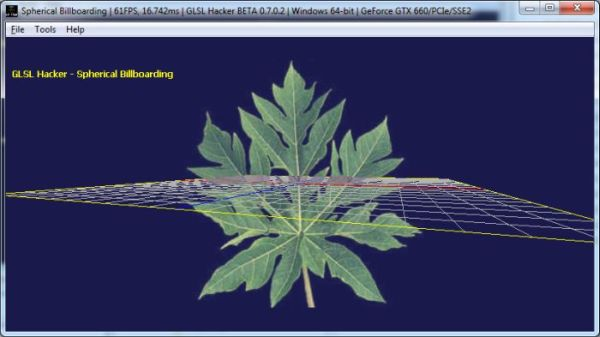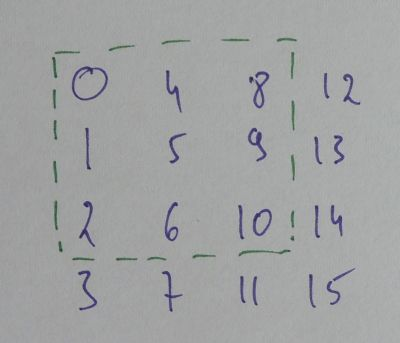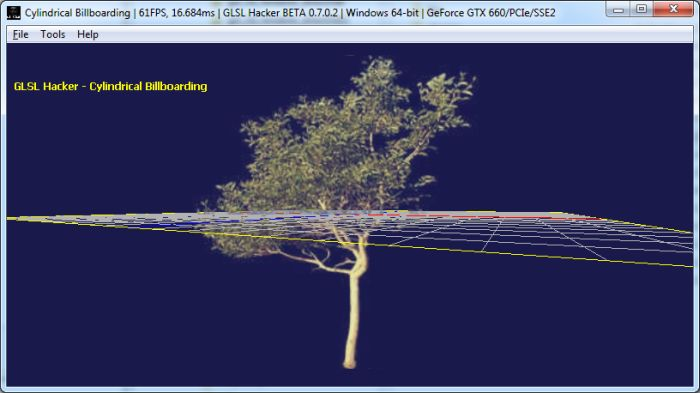# Simple Billboarding Vertex Shader (GLSL)Spherical billboarding

 Billboarding is a popular technique used in 3D graphics programming. Billboarding allows an object (usually a quad) to always face a given camera. Here are some common uses of billboarding: – particles – halo surrounding an object – trees rendering For the particular case of particles, the billboarding is a GPU built-in feature when point-sprites are used (a single point is transformed to a billboarded quad). The rest of this article will show two simple techniques (spherical and cylindrical) to do billboarding in the vertex shader without point-sprites.

Both techniques are similar and consist in tweaking the rotational part of the ModelView matrix. This matrix is the result of the multiplication of the View matrix (camera) and the Model matrix (object):

```mat4 ModelView = View * Model;
```

where mat4 is a GLSL 4×4 matrix.The rotation matrix included in the ModelView matrix is a 3×3 matrix (green dotted lines). This rotation matrix (three columns) can be extracted from the ModelView matrix like this:

```// Column 0:
ModelView  // 0
ModelView  // 1
ModelView  // 2

// Column 1:
ModelView  // 4
ModelView  // 5
ModelView  // 6

// Column 2:
ModelView  // 8
ModelView  // 9
ModelView  // 10
```

Spherical billboarding makes the object to always face the camera no matter the position of the camera on an imaginary sphere. Cylindrical billboarding makes the object to face the camera only when the camera looks at the right or at the left.

To do spherical billboarding, just remove all rotations by setting the identity matrix:

```// Column 0:
ModelView = 1;
ModelView = 0;
ModelView = 0;

// Column 1:
ModelView = 0;
ModelView = 1;
ModelView = 0;

// Column 2:
ModelView = 0;
ModelView = 0;
ModelView = 1;
```

If we don’t touch the second column (column 1), we get the cylindrical billboarding:

```// Column 0:
ModelView = 1;
ModelView = 0;
ModelView = 0;

// Column 2:
ModelView = 0;
ModelView = 0;
ModelView = 1;
```

## Cylindro-Spherical Billboarding GLSL Vertex Shader

Here is the complete GLSL vertex shader that performs the cylindro-spherical billboarding as it is used in the GLSL Hacker demos:

```#version 150
in vec4 gxl3d_Position;
in vec4 gxl3d_TexCoord0;

// GLSL Hacker automatic uniforms:
uniform mat4 gxl3d_ModelViewProjectionMatrix;
uniform mat4 gxl3d_ModelViewMatrix;
uniform mat4 gxl3d_ProjectionMatrix;
uniform mat4 gxl3d_ViewMatrix;
uniform mat4 gxl3d_ModelMatrix;

uniform int spherical; // 1 for spherical; 0 for cylindrical

out vec4 Vertex_UV;
void main()
{
//mat4 modelView = gxl3d_ViewMatrix*gxl3d_ModelMatrix;
mat4 modelView = gxl3d_ModelViewMatrix;

// First colunm.
modelView = 1.0;
modelView = 0.0;
modelView = 0.0;

if (spherical == 1)
{
// Second colunm.
modelView = 0.0;
modelView = 1.0;
modelView = 0.0;
}

// Thrid colunm.
modelView = 0.0;
modelView = 0.0;
modelView = 1.0;

vec4 P = modelView * gxl3d_Position;
gl_Position = gxl3d_ProjectionMatrix * P;

Vertex_UV = gxl3d_TexCoord0;
}
```

GLSL Hacker demos about billboarding are available in the host_api/GLSL_Billboarding/ folder of the code sample pack.Cylindrical billboarding

## One thought on “Simple Billboarding Vertex Shader (GLSL)”

1.Aily

Great!!! It works like a charm!

for HLSL matrices spherical sprite is same, but cylimdrical is:

M = 1;
//M = 0;
M = 0;

M = 0;
//M = 1;
M = 0;

M = 0;
//M = 0;
M = 1;